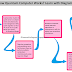A quantum computer is a special type of computer that uses the principles of quantum mechanics, quantum magic, superposition theory, and entanglement theory for its operation. Unlike classical computers that use bits to store and process information, quantum computers use quantum bits, or qubits, which can exist in multiple states simultaneously. This unique characteristic allows quantum computers to perform certain calculations much faster than classical computers. Quantum computers have the potential to solve complex problems in areas such as cryptography, optimization, drug discovery, and scientific simulations, offering exciting possibilities for advancements in technology and science. In this article, we will learn the step-by-step procedure of the working principle of a quantum computer with a block diagram. The block diagram helps to simplify the working concept so we can understand it easily.

Click on the Image to Enlarge

As you see in the above block diagram, the quantum computer works as below,
1. Unlike regular Os and 1s, quantum computers use Quantum Bits or Qubits that can be both 0 and 1 at the same time. This property is called superposition.
2. While Superposition makes the qubits be in multiple states simultaneously, the entanglement links qubits together so that the state of one qubit affects the others. It is called Quantum Magic.
3. Quantum Computer needs Quantum algorithms or instructions to tell the qubits how to perform calculations.
4. When we provide an instruction or algorithm to a quantum computer, it will perform calculations on the qubits according to those instructions. However, unlike regular computers that give exact results, quantum computers provide probabilistic results.
5. The Quantum Error Correction technique is used to eliminate errors and ensure accurate results.
6. Quantum computers solve problems very fast because they can perform multiple calculations simultaneously using Quantum Magic.

Quantum computers provide probabilistic results because of the inherent nature of quantum mechanics. In quantum computing, qubits can exist in a superposition of states, meaning they can be both 0 and 1 at the same time. So, when we perform calculations on qubits, the result is a combination of all possible states the qubits can be in. Due to this superposition, when we measure the qubits at the end of a computation, we obtain a probabilistic outcome. Each possible state has a certain probability of being observed as the final result. The probabilities are determined by the amplitudes associated with the different states in the superposition.

How Quantum Computer Works? Learn with DiagramReviewed by Author on June 21, 2023 Rating: 5